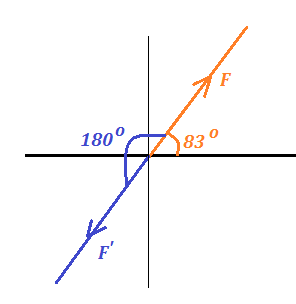# Given the vector F = 18N, \theta = 83 degrees, find the magnitude and direction of the opposite...

## Question:

Given the vector F = 18N, {eq}\theta {/eq} = 83 degrees, find the magnitude and direction of the opposite vector F.

## Resolution of Vectors:

Let the vector {eq}\vec A {/eq} is directed at an angle {eq}\theta {/eq} from the x-axis. Then we can write the vector as a sum of its components along the x- axis and the y-axis as in the following expression:

• {eq}\vec A = (|\vec A| \cos \theta) \hat i +(|\vec A| \sin \theta )\hat j {/eq}

where:

• {eq}|\vec A| {/eq} is the magnitude of the vector.

Given:

• The force vector is F = 18 N, it make an angle {eq}\theta = 83^o {/eq} from the +X axis.The opposite vector will have same magnitude ie F' = 18 N.

The angle of the opposite vector is {eq}\theta ' = (180+83)^o = 263^o {/eq} from the +X axis.